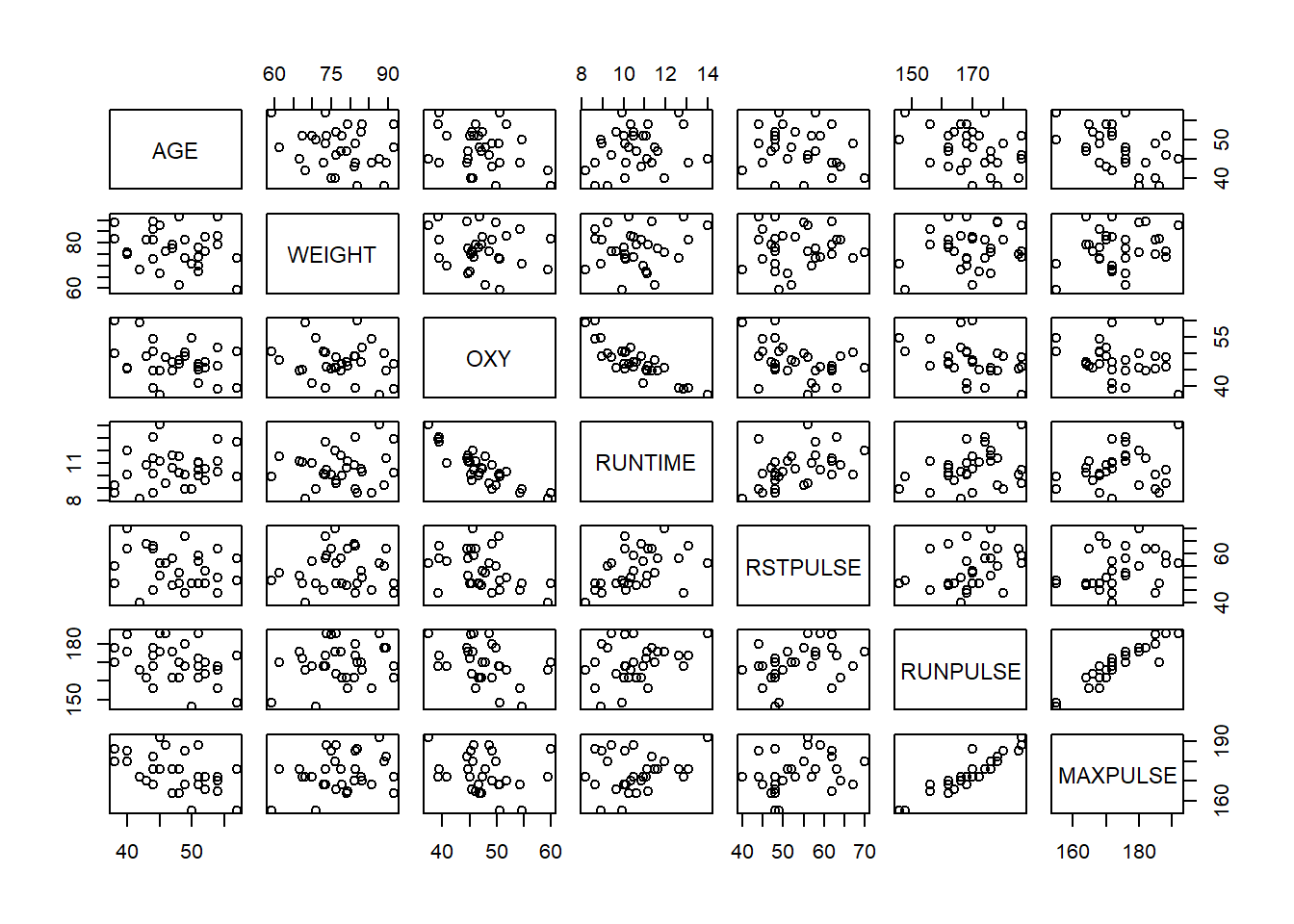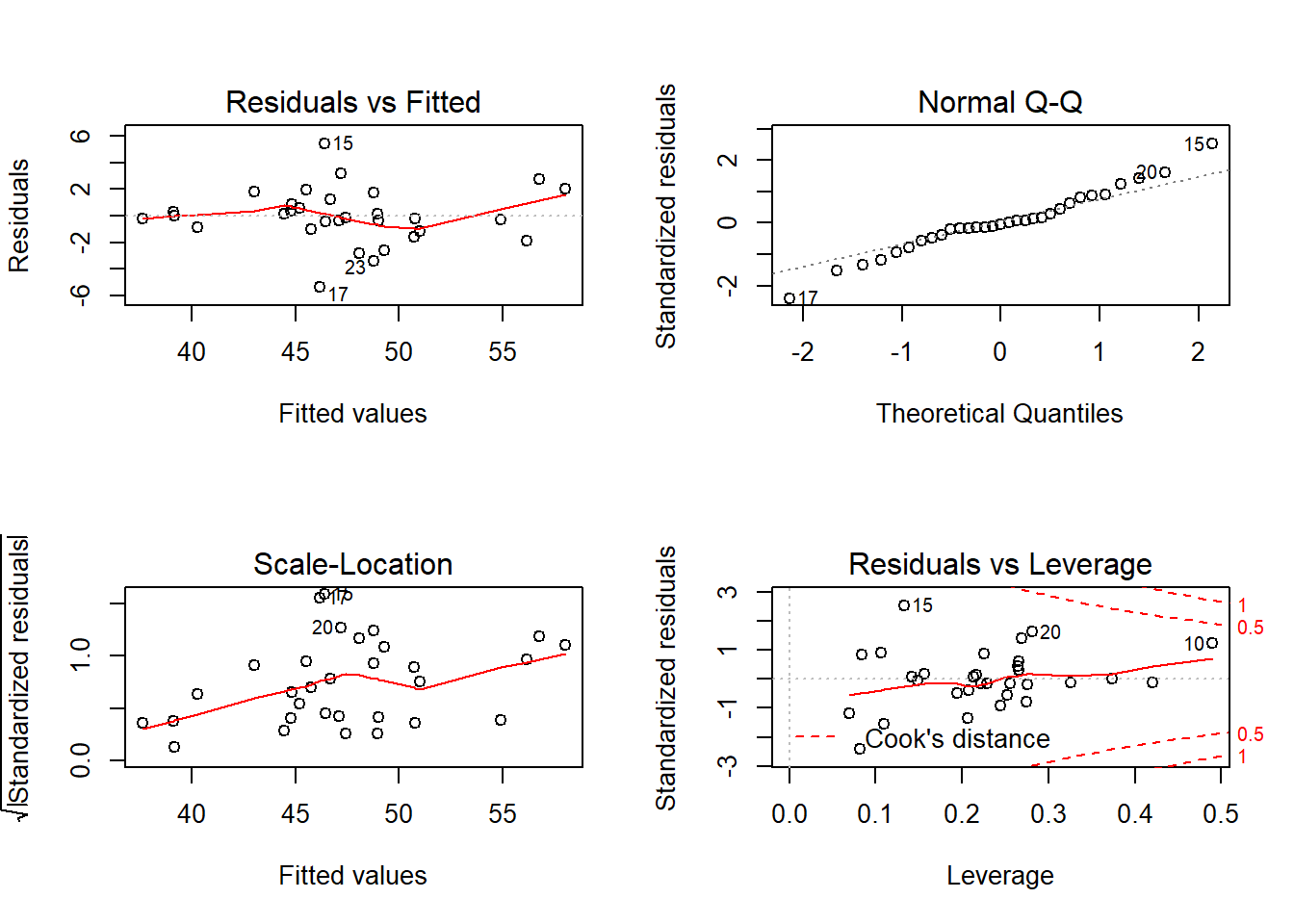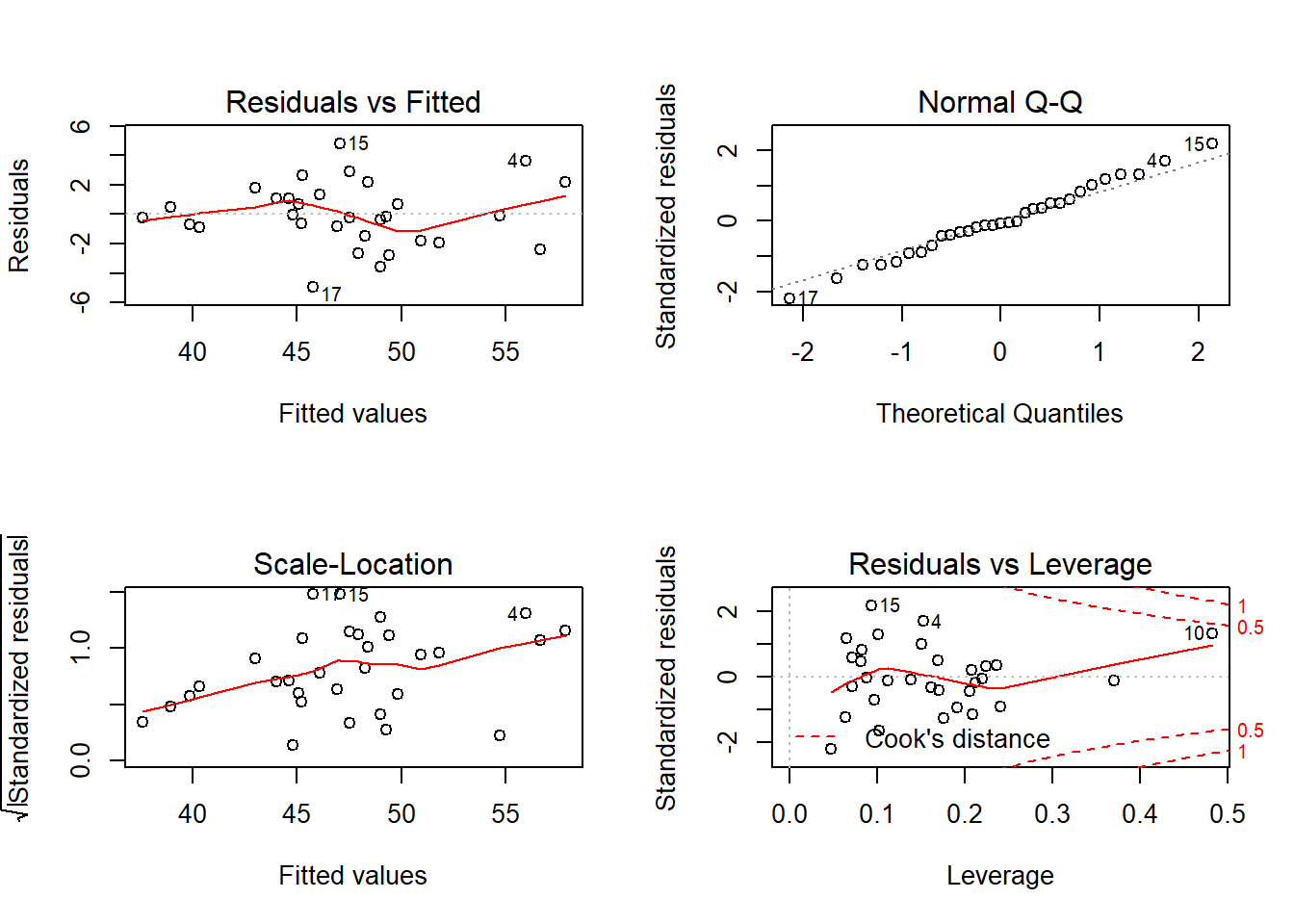# Introduction

For this study, we will be examining a sample composed of 31 runners. Our goal here is to attempt to consider the relationship between the consumption of oxygen by these runners according to several different aerobic fitness attributes of each runner. We are searching for a relationship between one or possibly several of these predictor variables with oxygen consumption, thus our analysis is inferential in nature. For this analysis, we will utilize the techniques of a multiple linear regression model to expose these relationships. We will test each fitness attribute according to the protocol of regression diagnostics in search of a suitable model for the data, if a relationship even exists. The data were gathered in a NC State University study of the same consideration of our data analysis of the effects of various aerobic fitness attributes on the oxygen consumption of runners. At the conclusion of our study, we found that the most prominent predictors providing the strongest relationship to oxygen consumption among runners are: the age of the runner, the pulse rate of the runner reached at the completion of their run, and the time taken for the runner to run 1.5 miles.

# Data

The response variable for our multiple linear regression model is the oxygen consumption in volume per unit body weight per unit time meaning any variable related to weight or time will be accounted for as part of the response. The variables to be included in the analysis are:
AGE = age in years
WEIGHT = weight in kilograms
OXY = oxygen consumption in volume per unit body weight per unit time
RUNTIME = time to run 1.5 miles
RSTPULSE = pulse rate at rest
RUNPULSE = pulse rate at end of run
MAXPULSE = maximum pulse rate during run

The data were obtained from a NC State University study. Upon examination of all 31 observations of the 7 variables including the response, it was determined that there were no apparent errors in the recording of the data. There were also no missing values in the data. The following is a brief numerical summary of each variable to familiarize the reader with the data and the expected numeric range of each variable.

fitness = read.table(file.choose(), sep="\t", header=T)
summary(fitness)
##       AGE            WEIGHT           OXY           RUNTIME
##  Min.   :38.00   Min.   :59.08   Min.   :37.39   Min.   : 8.17
##  1st Qu.:44.00   1st Qu.:73.20   1st Qu.:44.96   1st Qu.: 9.78
##  Median :48.00   Median :77.45   Median :46.77   Median :10.47
##  Mean   :47.58   Mean   :77.45   Mean   :47.38   Mean   :10.59
##  3rd Qu.:51.00   3rd Qu.:82.33   3rd Qu.:50.13   3rd Qu.:11.27
##  Max.   :57.00   Max.   :91.63   Max.   :60.05   Max.   :14.03
##     RSTPULSE        RUNPULSE        MAXPULSE
##  Min.   :40.00   Min.   :146.0   Min.   :155.0
##  1st Qu.:48.00   1st Qu.:163.0   1st Qu.:168.0
##  Median :52.00   Median :170.0   Median :172.0
##  Mean   :53.45   Mean   :169.6   Mean   :173.8
##  3rd Qu.:58.50   3rd Qu.:176.0   3rd Qu.:180.0
##  Max.   :70.00   Max.   :186.0   Max.   :192.0

Upon a quick examination of the summary statistics and running a simple Anderson-Darling normality test on each variable, each variable is satisfyingly derived from a normal distribution meaning the data should be more predictable and easier to work with. Each variable is a continuous variable meaning we will not have to account for any categorical variables within our data analysis.

# Analysis

Let us first take a look at the summary of each variable. By using str() function in R, we are able to see the structure of the fitness dataset.

str(fitness)
## 'data.frame':    31 obs. of  7 variables:
##  $AGE : int 44 40 44 42 38 47 40 43 44 38 ... ##$ WEIGHT  : num  89.5 75.1 85.8 68.2 89 ...
##  $OXY : num 44.6 45.3 54.3 59.6 49.9 ... ##$ RUNTIME : num  11.37 10.07 8.65 8.17 9.22 ...
##  $RSTPULSE: int 62 62 45 40 55 58 70 64 63 48 ... ##$ RUNPULSE: int  178 185 156 166 178 176 176 162 174 170 ...
##  \$ MAXPULSE: int  182 185 168 172 180 176 180 170 176 186 ...

Looks like we have all quantitative variables.

Now let us get a summary of each variable using the summary() function:

summary(fitness)
##       AGE            WEIGHT           OXY           RUNTIME
##  Min.   :38.00   Min.   :59.08   Min.   :37.39   Min.   : 8.17
##  1st Qu.:44.00   1st Qu.:73.20   1st Qu.:44.96   1st Qu.: 9.78
##  Median :48.00   Median :77.45   Median :46.77   Median :10.47
##  Mean   :47.58   Mean   :77.45   Mean   :47.38   Mean   :10.59
##  3rd Qu.:51.00   3rd Qu.:82.33   3rd Qu.:50.13   3rd Qu.:11.27
##  Max.   :57.00   Max.   :91.63   Max.   :60.05   Max.   :14.03
##     RSTPULSE        RUNPULSE        MAXPULSE
##  Min.   :40.00   Min.   :146.0   Min.   :155.0
##  1st Qu.:48.00   1st Qu.:163.0   1st Qu.:168.0
##  Median :52.00   Median :170.0   Median :172.0
##  Mean   :53.45   Mean   :169.6   Mean   :173.8
##  3rd Qu.:58.50   3rd Qu.:176.0   3rd Qu.:180.0
##  Max.   :70.00   Max.   :186.0   Max.   :192.0

## Analysis

We will first plot a matrix plot of all the variables. Let us use the pairs() function.

pairs(fitness)We see from first glance that runpulse and max pulse are strongly correlated. In addition, oxy and runtime appear to be negatively correlated.

Let us checkout a basic model by regressing oxygeon on the remaining variables:

fitness.lm = lm(OXY~., data = fitness)
summary(fitness.lm)
##
## Call:
## lm(formula = OXY ~ ., data = fitness)
##
## Residuals:
##     Min      1Q  Median      3Q     Max
## -5.3589 -0.9241 -0.1396  1.0343  5.4336
##
## Coefficients:
##              Estimate Std. Error t value Pr(>|t|)
## (Intercept) 104.03396   12.74920   8.160 2.22e-08 ***
## AGE          -0.23362    0.10280  -2.273   0.0323 *
## WEIGHT       -0.07501    0.05461  -1.374   0.1823
## RUNTIME      -2.59095    0.39067  -6.632 7.36e-07 ***
## RSTPULSE     -0.02365    0.06623  -0.357   0.7242
## RUNPULSE     -0.37391    0.11964  -3.125   0.0046 **
## MAXPULSE      0.30149    0.13671   2.205   0.0373 *
## ---
## Signif. codes:  0 '***' 0.001 '**' 0.01 '*' 0.05 '.' 0.1 ' ' 1
##
## Residual standard error: 2.316 on 24 degrees of freedom
## Multiple R-squared:  0.8488, Adjusted R-squared:  0.811
## F-statistic: 22.46 on 6 and 24 DF,  p-value: 9.597e-09

From the model summary, we see a few things. It seems that the only variables that are significant at a 95% confidence level are RunTime, RunPulse, and MaxPulse. Our F-stat has a p-value of 9.597e-09, which means we reject the null hypothesis and conclude that at least one of our coefficients is statistically not equal to zero. Our RSE is 2.316. The adjusted R-squared is 0.811, so our model does a decent job at modeling Oxygen consumption.

Let us conduct some diagnostics and check some assumptions for this model.

We will need to check diagnostic plots of our residuals.
Some common problems of regressions:
1. Non-linearity of the response-predictor relationships.
2. Correlation of error terms.
3. Non-constant variance of error terms.
4. Outliers.
5. High-leverage points.
6. Collinearity.

Let us graph the residual plots:

par(mfrow=c(2,2))
plot(fitness.lm)From the above plots, we see that our residuals appear to have a linear pattern which is a good sign. In addition, our residuals appear to be from a normal distribution, because the points on the qq plot stay close to the line for the most part. Although there is not any clear ‘funneling’ or ‘fanning’ of our residuals, they appear to have more variance in the middle and are close to zero at the tails. Also, it seems that we have some possible outliers and maybe some high leverage points. There are issues with points: 10, 15, 17, 20, and 23.

We will now investigate the variables for any multi-collinearity. Will utilize the ‘car’ library and the VIF function. Let us install and import the library.

##install.packages('car')
library(car)
## Loading required package: carData

Now let us run the VIF function on our model:

vif(fitness.lm)
##      AGE   WEIGHT  RUNTIME RSTPULSE RUNPULSE MAXPULSE
## 1.607005 1.154878 1.643502 1.424733 8.415728 8.780650

We see that our VIF’s appear to be low to moderate and there is nothing that indicates serious problems.

Let us summarize what we could improve on this model:
- We can get rid of insignificant variables.
- Can get rid of some outliers and high leverage points.

## Updated Model

Let us first fit the model without the variables that were not significant, which were weight and restpulse.

fitness.lm2 = lm(OXY~.-WEIGHT - RSTPULSE, data = fitness)
summary(fitness.lm2)
##
## Call:
## lm(formula = OXY ~ . - WEIGHT - RSTPULSE, data = fitness)
##
## Residuals:
##     Min      1Q  Median      3Q     Max
## -4.9519 -1.1952 -0.1651  1.2233  4.8097
##
## Coefficients:
##             Estimate Std. Error t value Pr(>|t|)
## (Intercept) 98.99164   12.10182   8.180 1.16e-08 ***
## AGE         -0.20255    0.09829  -2.061   0.0495 *
## RUNTIME     -2.74046    0.34480  -7.948 2.00e-08 ***
## RUNPULSE    -0.35196    0.11736  -2.999   0.0059 **
## MAXPULSE     0.26897    0.13395   2.008   0.0551 .
## ---
## Signif. codes:  0 '***' 0.001 '**' 0.01 '*' 0.05 '.' 0.1 ' ' 1
##
## Residual standard error: 2.313 on 26 degrees of freedom
## Multiple R-squared:  0.8367, Adjusted R-squared:  0.8115
## F-statistic:  33.3 on 4 and 26 DF,  p-value: 6.994e-10

Here we have an adjusted R-squared of 0.8115 which is slightly better than the first model, this is good because we used less variables.

Let us gnow checkout our residual plots:

par(mfrow=c(2,2))
plot(fitness.lm2)We still seem to have some outliers and high leverage points.

Let us remove point 10 as it seems to possibly be a high leveraged point because the Cook’s distance graph has that point listed a little high. First create a new dataset as to not mess up our original data set.

fitness2 = fitness[-c(10),]

Now we have data without observation #10 which seemed to be a high leverage point. Let us see if it made a difference in our regression.

fitness.lm3 = lm(OXY~.-WEIGHT - RSTPULSE, data = fitness2)
summary(fitness.lm3)
##
## Call:
## lm(formula = OXY ~ . - WEIGHT - RSTPULSE, data = fitness2)
##
## Residuals:
##     Min      1Q  Median      3Q     Max
## -4.9559 -1.1647  0.0555  1.0791  4.7527
##
## Coefficients:
##              Estimate Std. Error t value Pr(>|t|)
## (Intercept) 101.73559   12.08543   8.418 9.17e-09 ***
## AGE          -0.18511    0.09762  -1.896   0.0695 .
## RUNTIME      -2.66673    0.34380  -7.757 4.11e-08 ***
## RUNPULSE     -0.23990    0.14220  -1.687   0.1040
## MAXPULSE      0.13372    0.16552   0.808   0.4268
## ---
## Signif. codes:  0 '***' 0.001 '**' 0.01 '*' 0.05 '.' 0.1 ' ' 1
##
## Residual standard error: 2.277 on 25 degrees of freedom
## Multiple R-squared:  0.8109, Adjusted R-squared:  0.7806
## F-statistic:  26.8 on 4 and 25 DF,  p-value: 1.013e-08

Seems that made runpulse, maxpulse, and age insignificant variables. Let us see what happens if we remove those variables as well.

fitness.lm4 = lm(OXY~.-WEIGHT - RSTPULSE-RUNPULSE - MAXPULSE- AGE, data = fitness2)
summary(fitness.lm4)
##
## Call:
## lm(formula = OXY ~ . - WEIGHT - RSTPULSE - RUNPULSE - MAXPULSE -
##     AGE, data = fitness2)
##
## Residuals:
##     Min      1Q  Median      3Q     Max
## -5.1980 -1.4773  0.0055  1.6004  4.9816
##
## Coefficients:
##             Estimate Std. Error t value Pr(>|t|)
## (Intercept)  79.7322     3.6675  21.740  < 2e-16 ***
## RUNTIME      -3.0775     0.3416  -9.008 9.16e-10 ***
## ---
## Signif. codes:  0 '***' 0.001 '**' 0.01 '*' 0.05 '.' 0.1 ' ' 1
##
## Residual standard error: 2.506 on 28 degrees of freedom
## Multiple R-squared:  0.7435, Adjusted R-squared:  0.7343
## F-statistic: 81.15 on 1 and 28 DF,  p-value: 9.164e-10

Nope, seems that it made our model worse a little.
We will undo removing point 10 and continue with our model before removing it.

It seems that RUNPULSE and MAXPULSE are correlated a little, so we will drop one of the and see if our model is better.

fitness.lm5=lm(OXY~AGE+ RUNTIME+ RUNPULSE, data = fitness)
summary(fitness.lm5)
##
## Call:
## lm(formula = OXY ~ AGE + RUNTIME + RUNPULSE, data = fitness)
##
## Residuals:
##     Min      1Q  Median      3Q     Max
## -4.8510 -1.2858  0.1432  1.0664  4.9543
##
## Coefficients:
##              Estimate Std. Error t value Pr(>|t|)
## (Intercept) 112.80814   10.49962  10.744 2.98e-11 ***
## AGE          -0.26351    0.09860  -2.673   0.0126 *
## RUNTIME      -2.78810    0.36279  -7.685 2.89e-08 ***
## RUNPULSE     -0.13781    0.05169  -2.666   0.0128 *
## ---
## Signif. codes:  0 '***' 0.001 '**' 0.01 '*' 0.05 '.' 0.1 ' ' 1
##
## Residual standard error: 2.439 on 27 degrees of freedom
## Multiple R-squared:  0.8113, Adjusted R-squared:  0.7904
## F-statistic:  38.7 on 3 and 27 DF,  p-value: 6.446e-10

That did not help. Will try an interaction variable between run and max pulse

fitness.lm6 = lm(OXY~AGE + RUNTIME + RUNPULSE*MAXPULSE, data = fitness)
summary(fitness.lm6)
##
## Call:
## lm(formula = OXY ~ AGE + RUNTIME + RUNPULSE * MAXPULSE, data = fitness)
##
## Residuals:
##    Min     1Q Median     3Q    Max
## -5.209 -1.206  0.051  1.252  4.521
##
## Coefficients:
##                     Estimate Std. Error t value Pr(>|t|)
## (Intercept)        18.261496 112.549719   0.162   0.8724
## AGE                -0.186981   0.101530  -1.842   0.0774 .
## RUNTIME            -2.771644   0.350700  -7.903 2.93e-08 ***
## RUNPULSE            0.101338   0.639297   0.159   0.8753
## MAXPULSE            0.750623   0.681081   1.102   0.2809
## RUNPULSE:MAXPULSE  -0.002715   0.003763  -0.722   0.4773
## ---
## Signif. codes:  0 '***' 0.001 '**' 0.01 '*' 0.05 '.' 0.1 ' ' 1
##
## Residual standard error: 2.334 on 25 degrees of freedom
## Multiple R-squared:   0.84,  Adjusted R-squared:  0.808
## F-statistic: 26.25 on 5 and 25 DF,  p-value: 3.395e-09

Seems that a better model than a model fit with all the varialbes one with weight and rest pulse. Our adjusted R-squared is only slight better than the original model.

All in all, it seems that we had some redundant variables so removing those seemed to help a little. We maybe could have removed some of the other outliers/high leverage points and seeing if they made a difference.